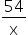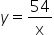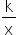Mathematics
Easy

Question

# Given the following equation, identify the constant k. y =## There is one k = x54 y = kHint:

## The correct answer is: 54

### Algebra is a subfield of mathematics that deals with symbols, variables, numbers, and the rules for their manipulation. It states that expressions and equations are created using the mathematical relationship to find the unknown number.Unknown variables, constants, and coefficients are used to represent these expressions. These three terms combined are referred to as an expression. It should be noted that an algebraic expression lacks sides and the equal sign, in contrast to an algebraic equation.Example: 3x + 4y – 7,  4x – 10A constant is a value or number that is consistently the same regardless of how it is expressed.A variable is a letter that stands in for an unknown; it always represents a number but can take on several meanings when used in an expression. Mathematicians typically designate letters (not necessarily) toward the end of the alphabet (such as x, y, and z) as variables.Here we have given the expression as, soy =and here k = 54So the constant is 54.

Here we understood the concept of algebriac expressions, what are the terms involved in it like constants, variables, etc which are the major aspect of an expression. So here the expression was. So the constant is 54.

### Related Questions to study#### With Turito Foundation.#### Get an Expert Advice From Turito.